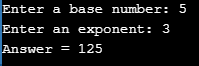# How To Best Utilize Power Function In C?

Published on Sep 10,2019 14.5K ViewsFinding power of a number is a very common operation in programming logic. In this article we will understand how to write a program by using Power Function In C to calculate power of a number. Following pointers will be covered in this article,

Let us start with understanding how to write a program to calculate power of a number.

## Logic For Power Multiplication

23 = 2*2*2 = 8

To find the 2^3, you need to multiply 2 thrice. This can be simply achieved using for loop.

For above example, base = 2, exponent = 3.

```for (exponent=3; exponent>0; exponent--)
{
result = result * base;
}```

Let’s look at the C program implementing the same.

Example

```#include <stdio.h>
int main()
{
int base, exponent;
int result = 1;
printf("Enter a base number: ");
scanf("%d", &base);
printf("Enter an exponent: ");
scanf("%d", &exponent);
for (exponent; exponent>0; exponent--)
{
result = result * base;
}
return 0;
}```

Output:Moving On with this article on Power Function In C

## Writing a Custom Power Function

You can also create a function to calculate the power which you can call anytime you want to calculate the power of any integer.

```int power(int base, int exponent)
{
int result=1;
for (exponent; exponent>0; exponent--)
{
result = result * base;
}
return result;
}```

Let’s look at the complete code how to use this function.

Example

```#include <stdio.h>
int power(int base, int exponent){
int result=1;
for(exponent; exponent>0; exponent--){
result = result * base;
}
return result;
}
int main()
{
int base, exponent;
printf("Enter a base number: ");
scanf("%d", &base);
printf("Enter an exponent: ");
scanf("%d", &exponent);
int res = power(base, exponent);
return 0;
}```

Output:This technique only works if the exponent is a positive integer. If you want to find the power of a number where the exponent is a real number, you need to use pow() function.

## Power Function in C

• The pow() function is used to find the power of a given number.
• It returns x raised to the power of y(i.e. xy).
• The pow() function is present in math.h header file. So, you need to import math.h header file in the program to use pow() function.

#include <math.h>

• The declaration of the power function is as follows:

Declaration: double pow(double base, double exponent);

• The pow() function takes two arguments (i.e. base & exponent) & returns the result of raising base to the power exponent.
• Calling the power function:

pow(2.4, 3.2)

where 2.4 is the base & 3.2 is the exponent

Let’s quickly look at an example to understand how to use pow() function in a program.

Example:

```#include <stdio.h>
#include <math.h>
int main()
{
double base, expo, res;
printf("Enter a base number: ");
scanf("%lf", &base);
printf("Enter an exponent: ");
scanf("%lf", &expo);
res = pow(base, expo);
printf("%.1lf^%.1lf = %.2lf", base, expo, res);
return 0;
}```

OutputNow, after executing the above programs you would have understood how to use power function in C. I hope this article was informative and added value to you.

Stay tuned for more tutorials on similar topics.You may also checkout our training program to get in-depth knowledge on jQuery along with its various applications, you can enroll here for live online training with 24/7 support and lifetime access.Implement the above code with different strings and modifications. Now, we have a good understanding of all key concepts related to the pointer.

Got a question for us? Mention them in the comments section of  this blog and we will get back to you.